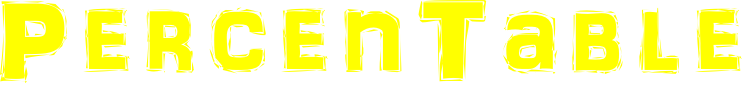### Copy and complete this table without using a calculator!

 180 600 1320 13260 10% 50% 25% 33⅓% 75% 90%

## A Mathematics Lesson Starter Of The Day

• Transum,
•
• Transum's exciting, fascinating and surprising starters are balanced by this more routine practice exercise. Having said that some pupils will find it a revelation when they learn (from other pupils) tricks for quickly working out these common percentages. A really useful way to spend the first ten minutes of the lesson.
• Dylan T, Charnwood Primary Lichfield
•
• This was a good starter especially if your target is percentages!
• Faye D Year 6, Charnwood Primary Lichfield
•
• A good starter as it got my brain working and lots of people found it challenging.
• Transum,
•
• HELP! How do you convert a fraction to a percentage? Do you multiply by 100 or do you multiply by 100% ? Textbooks differ and I have been having a conversation which is leaving me undecided. You can see the discussion here

How did you use this starter? Can you suggest how teachers could present or develop this resource? Do you have any comments? It is always useful to receive feedback and helps make this free resource even more useful for Maths teachers anywhere in the world.

Previous Day | This starter is for 16 October | Next Day

% - This is the percent symbol.
Percent means 'out of 100'.

### Find 50%:

As 50 is half of 100, then 50% means half. To find 50% of a quantity you need to halve (or divide by two). So 50% of 6 is 3.

### Find 10%:

As 10 is one tenth of 100, then 10% means 'one tenth of'. To find 10% of a quantity you need to divide it by ten. So 10% of 800 is 80.

### Find 25%:

As 25 is one quarter of 100, then 25% means 'one quarter of'. To find 25% of a quantity you need to divide it by four. So 25% of 20 is 5.

Another way of finding 25% of a quantity is first finding 50% then dividing the result by 2.

### Find 33⅓%:

As 33⅓ is one third of 100, then 33⅓% means 'one third of'. To find 33⅓% of a quantity you need to divide it by three. So 33⅓% of 30 is 10.

### Find 1%:

As 1 is one hundredth of 100, then 1% means 'one hundredth of'. To find 1% of a quantity you need to divide it by 100. So 1% of 800 is 8.

### Find other percentages:

Other percentages can be found by combining some of the techniques mentioned above. Here are some examples:

• To find 75% of a quantity add together 50% and 25% of it.
• To find 20% of a quantity double 10% of it.
• To find 5% of a quantity halve 10% of it.
• To find 66⅔% of a quantity double 33⅓% of it.
• To find 90% of a quantity subtract 10% from it.
• To find 80% of a quantity subtract 20% from it.
• To find 2% of a quantity double 1% of it.
• To find 52% of a quantity add 50% to 2% of it.

If you need to use a calculator to check your working. See Calculator Workout skill 3.

### Commute

Did you know that if you are struggling to mentally work out 24% of 50 you can switch the numbers round and work out 50% of 24 instead. Finding 50% is very easy isn’t it? You will get the same answer.

Finding a percentage of a quantity is an example of a commutative calculation. Not all operations are commutative. Subtraction certainly isn’t as 10 minus one is not the same as one minus ten.

You can use this trick to improve your ability to do this type of calculation quickly if you find the switch makes it easier.

Practise with 12% of 50, 4% of 25 and 75% of 10.

 180 600 1320 13260 10% 18 60 132 1326 50% 90 300 660 6630 25% 45 150 330 3315 33⅓% 60 200 440 4420 75% 135 450 990 9945 90% 162 540 1188 11934

Note to teacher: Doing this activity once with a class helps students develop strategies. It is only when they do this activity a second time that they will have the opportunity to practise those strategies. That is when the learning is consolidated. Click the button above to regenerate another version of this starter from random numbers.

Your access to the majority of the Transum resources continues to be free but you can help support the continued growth of the website by doing your Amazon shopping using the links on this page. Below is an Amazon search box and some items I have chosen and recommend to get you started. As an Amazon Associate I earn a small amount from qualifying purchases which helps pay for the upkeep of this website.Teacher, do your students have access to computers?Do they have iPads or Laptops in Lessons? Whether your students each have a TabletPC, a Surface or a Mac, this activity lends itself to eLearning (Engaged Learning).Transum.org/go/?Start=October16

Here is the URL which will take them to a related student activity.

Transum.org/go/?to=pchange## Curriculum Reference

See the National Curriculum page for links to related online activities and resources.For All: